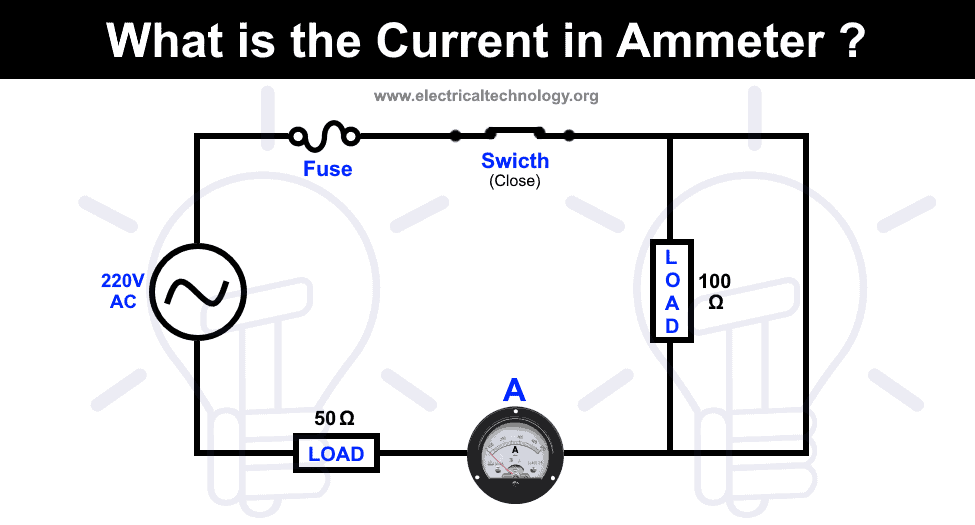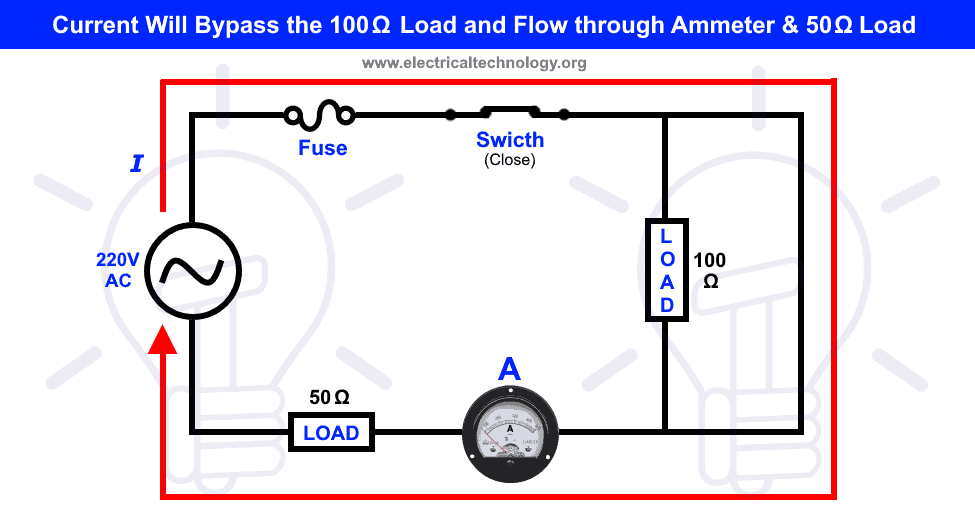# What is the Current in Ampere Meter?

## Ampere Meter Basic Concept: What is the Current in Ammeter ?

Basic Electrical Engineering Interview Question Series: An ammeter is connected in series with a 50 ohms and 100 ohms loads. If there is a short path across the 100 ohms load, what is the value of current in ampere meter?Short Answer: 4.4 Amperes as current is only passing through the 50 ohms load and ampere meter neglecting the 100 ohms load due to short path.

We know that current always choose a short and easiest way to flow through it. In other words, current always select the low resistance path in parallel circuit and if there is short passage, it will ignore the load connected in the circuit.

The same will happens in the above case i.e. current will ignore the 100 ohms load as there is a short path with almost zero resistance. So the flow of current is shown by blue line with arrow from the source to the load and ampere meter. In other words, current will flow through the ampere meter and 50 ohms load bypassing the 100 ohms load due to parallel short path across it.To know the value of flowing current through ammeter and 50 ohms load, we may use the basic Ohm’s law as follow.

I = V / R

Putting the values.

I = 220V / 50Ω …[ Neglecting the ampere meter internal resistance and 100 Ohm Resistance]

I = 4.4 Amperes.

So the total current flowing through the ammeter and load is 4.4A.

### Electrical Technology

The circuit tells us dead short circuit condition because Ammeter has very low internal resistance and the load has very high resistance (100 ohms). Always current flows through low resistance path. Very high current(220/Ra, ideally Ra=0, practically it is almost less than 0.18 ohms) flows through Ammeter but Ammeter connected in series with fuse( high resistance load will act as open circuit) due to very high current fuse will melt after switching On button and entire circuit become an open circuit then the current flows through circuit or Ammeter becomes zero.

2. Sneha says:

There will be no any current flow through the ammeter. Once the current starts to flow the circuit will be damaged because of parallel connection. Ammeter has low resistance. If current flow it will choose the lower resistive path that is ammeter. Due to this ammeter will be damage or burn.

1. Raz Rojas B says:

The ammeter’s low resistance will short the circuit. Assuming it is say 100mOhm, current will be 2200A, which will blow both the fuse in your circuit and the fuse in the ammeter, if it has one.